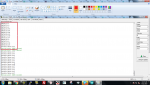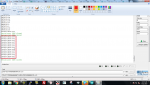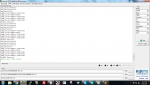# How to convert modbus 8byte hex to double

#### kannannatesh

oh sorry i forget to the device address, register address bytes, and length of the bytes, then also forget to write the j++ lines after the serial print function if i upload my above program to my Arduino definitely that will confusing me for some minutes so thanks for point out my mistakes, i will share my happiness after the successful conversion.
I was done all the correction in my code and the output is given below for your reference, and if i change register address 74 instead of 75 i am getting nothing so i don't change register address, here i have two problems why the "j"(unsigned int) value does not increase to greater than 9. then output line print ovf why?

#include <SoftwareSerial.h>

#define Pin13LED 13
byte byteSend;

unsigned short reg_data;
long temp_int64;
double final_value;
unsigned int j = 0;
byte request[] = {0x01, 0x03, 0x00, 0x75, 0x00, 0x04, 0x55, 0xD3};
void setup()
{
Serial.begin(9600);
Serial1.begin(9600);
Serial.println("MODBUS Test...");
}
void loop()
{
//Serial1.write(request, sizeof(request));
/*int i = 0;
for (i = 0; i < sizeof(request); i++)
{
Serial1.write(request);
//Serial1.print(request,BIN);
}*/
while (Serial1.available())
{
Serial.print("DATA[");
Serial.print(j,DEC);
Serial.print("]:");
Serial.println(byteSend[j], HEX);
j++;
}
reg_data = (((unsigned short)byteSend) << 8) | ((unsigned short)byteSend);
reg_data = (((unsigned short)byteSend) << 8) | ((unsigned short)byteSend);
reg_data = (((unsigned short)byteSend) << 8) | ((unsigned short)byteSend);
reg_data = (((unsigned short)byteSend) << 8) | ((unsigned short)byteSend);

// Copy the least significant 16-bit register
temp_int64 = (long)reg_data;

// Copy the next 16-bit register
temp_int64 = temp_int64 | (((long)reg_data) << 16);

// Copy the next 16-bit register
temp_int64 = temp_int64 | (((long)reg_data) << 32);

// Copy the most significant 16-bit register
temp_int64 = temp_int64 | (((long)reg_data) << 48);

// Convert the 64-bit integer to a double
final_value = *((double *)&temp_int64);

Serial.print("Double Data:");
Serial.println(final_value);
j = 0;
Serial1.write(request, sizeof(request));
delay(1000);
}

output:i have just change the code to check the conversion method so i add the values for byte read variable at default as below

byteSend =0x01;
byteSend =0x03;
byteSend =0x08;
byteSend =0xFC;
byteSend =0xF4;
byteSend =0xD4;
byteSend =0x82;
byteSend =0x3F;
byteSend =0xF7;
byteSend =0x00;
byteSend =0x03;
byteSend =0x39;
byteSend =0x25;

reg_data = (((unsigned short)byteSend) << 8) | ((unsigned short)byteSend);
reg_data = (((unsigned short)byteSend) << 8) | ((unsigned short)byteSend);
reg_data = (((unsigned short)byteSend) << 8) | ((unsigned short)byteSend);
reg_data = (((unsigned short)byteSend) << 8) | ((unsigned short)byteSend);

outputi can't understand the problem kindly help to understand what is going on here

#### kannannatesh

Hi I have print the values in my serial that is attached below with code it will help to understand the problem clearly

#include <SoftwareSerial.h>

#define Pin13LED 13
byte byteSend;

unsigned short reg_data;
long temp_int64;
double final_value;
unsigned int j = 0;
byte request[] = {0x01, 0x03, 0x00, 0x75, 0x00, 0x04, 0x55, 0xD3};
void setup()
{
Serial.begin(9600);
Serial1.begin(9600);
Serial.println("MODBUS Test...");
}
void loop()
{
//Serial1.write(request, sizeof(request));
/*int i = 0;
for (i = 0; i < sizeof(request); i++)
{
Serial1.write(request);
//Serial1.print(request,BIN);
}*/
while (Serial1.available())
{
Serial.print("DATA[");
Serial.print(j,DEC);
Serial.print("]:");
Serial.println(byteSend[j], HEX);
j++;
}

byteSend =0x01;
byteSend =0x03;
byteSend =0x08;
byteSend =0xFC;
byteSend =0xF4;
byteSend =0xD4;
byteSend =0x82;
byteSend =0x3F;
byteSend =0xF7;
byteSend =0x00;
byteSend =0x03;
byteSend =0x39;
byteSend =0x25;

reg_data = (((unsigned short)byteSend) << 8) | ((unsigned short)byteSend);
Serial.print("reg_data=");
Serial.println(reg_data);
reg_data = (((unsigned short)byteSend) << 8) | ((unsigned short)byteSend);
Serial.print("reg_data=");
Serial.println(reg_data);
reg_data = (((unsigned short)byteSend) << 8) | ((unsigned short)byteSend);
Serial.print("reg_data=");
Serial.println(reg_data);
reg_data = (((unsigned short)byteSend) << 8) | ((unsigned short)byteSend);
Serial.print("reg_data=");
Serial.println(reg_data);

Serial.print("temp_int64 STEP0=");
Serial.println(temp_int64);
// Copy the least significant 16-bit register
temp_int64 = (long)reg_data;
Serial.print("temp_int64 STEP1=");
Serial.println(temp_int64);
// Copy the next 16-bit register
temp_int64 = temp_int64 | (((long)reg_data) << 16);
Serial.print("temp_int64 STEP2=");
Serial.println(temp_int64);
// Copy the next 16-bit register
temp_int64 = temp_int64 | (((long)reg_data) << 32);
Serial.print("temp_int64 STEP3=");
Serial.println(temp_int64);
// Copy the most significant 16-bit register
temp_int64 = temp_int64 | (((long)reg_data) << 48);

Serial.print("temp_int64 STEP4=");
Serial.println(temp_int64);
// Convert the 64-bit integer to a double
final_value = *((double *)&temp_int64);

Serial.print("Double Data:");
Serial.println(final_value);
j = 0;
// Serial1.write(request, sizeof(request));
delay(1000);
}

output:#### jschulze

Three main problems.

1. You absolutely need to use 74 instead of 75 for the address. The reason you're not getting a response is because you haven't recalculated your CRC when you changed 75 to 74. The entire packet should be this:
{0x01, 0x03, 0x00, 0x74, 0x00, 0x04, 0x04, 0x13}

Please double-check that the CRC I calculated is correct.

This is likely also the reason you're seeing the "Double Data: ovf" in your output, because you're reading the wrong registers from the sensor the hex data you're using is incorrect. Compare the hex values you see with your program to what you successfully read using ModScan.

2. You are overrunning your byteSend buffer. You have it set to a size of 10, but the response packet from your sensor will be 13 bytes (slave address, function code, byte count, 4 register values, CRC).

3. You should move your delay(1000); line so that it is before the while (Serial1.available()). Otherwise, the while loop may be skipped because the sensor has not had time to respond.

#### kannannatesh

Three main problems.

1. You absolutely need to use 74 instead of 75 for the address. The reason you're not getting a response is because you haven't recalculated your CRC when you changed 75 to 74. The entire packet should be this:
{0x01, 0x03, 0x00, 0x74, 0x00, 0x04, 0x04, 0x13}

Please double-check that the CRC I calculated is correct.

This is likely also the reason you're seeing the "Double Data: ovf" in your output, because you're reading the wrong registers from the sensor the hex data you're using is incorrect. Compare the hex values you see with your program to what you successfully read using ModScan.

2. You are overrunning your byteSend buffer. You have it set to a size of 10, but the response packet from your sensor will be 13 bytes (slave address, function code, byte count, 4 register values, CRC).

3. You should move your delay(1000); line so that it is before the while (Serial1.available()). Otherwise, the while loop may be skipped because the sensor has not had time to respond.
oh sorry, i will recheck the code and update on Monday then i will share the output, i will check the CRC value using the Modbus software, thanks for point out my mistakes

#### kannannatesh

oh sorry, i will recheck the code and update on Monday then i will share the output, i will check the CRC value using the Modbus software, thanks for point out my mistakes
Hi, i changed everything that you suggest in previous, but i am getting an error in double, the CRC value is correct that is shown in COm test pro. jpg, the output data is shown in Hercules.jpg, Hex data from Modbus is shown in Modscan.jpg
my code is shown in below

#include <SoftwareSerial.h>

#define Pin13LED 13
byte byteSend;

unsigned short reg_data;
long temp_int64;
double final_value;
unsigned int j = 0;
byte request[] = {0x01, 0x03, 0x00, 0x74, 0x00, 0x04, 0x04, 0x13};
//{0x01, 0x03, 0x00, 0x74, 0x00, 0x04, 0x04, 0x13}
//{0x01, 0x03, 0x00, 0x75, 0x00, 0x04, 0x55, 0xD3};
void setup()
{
Serial.begin(9600);
Serial1.begin(9600);
Serial.println("MODBUS Test...");
}
void loop()
{
Serial1.write(request, sizeof(request));
delay(1000);
while (Serial1.available())
{
Serial.print("DATA[");
Serial.print(j,DEC);
Serial.print("]:");
Serial.println(byteSend[j], HEX);
j++;
}

reg_data = (((unsigned short)byteSend) << 8) | ((unsigned short)byteSend);
Serial.print("reg_data=");
Serial.println(reg_data);
reg_data = (((unsigned short)byteSend) << 8) | ((unsigned short)byteSend);
Serial.print("reg_data=");
Serial.println(reg_data);
reg_data = (((unsigned short)byteSend) << 8) | ((unsigned short)byteSend);
Serial.print("reg_data=");
Serial.println(reg_data);
reg_data = (((unsigned short)byteSend) << 8) | ((unsigned short)byteSend);
Serial.print("reg_data=");
Serial.println(reg_data);

Serial.print("temp_int64 STEP0=");
Serial.println(temp_int64);
// Copy the least significant 16-bit register
temp_int64 = (long)reg_data;
Serial.print("temp_int64 STEP1=");
Serial.println(temp_int64);
// Copy the next 16-bit register
temp_int64 = temp_int64 | (((long)reg_data) << 16);
Serial.print("temp_int64 STEP2=");
Serial.println(temp_int64);
// Copy the next 16-bit register
temp_int64 = temp_int64 | (((long)reg_data) << 32);
Serial.print("temp_int64 STEP3=");
Serial.println(temp_int64);
// Copy the most significant 16-bit register
temp_int64 = temp_int64 | (((long)reg_data) << 48);

Serial.print("temp_int64 STEP4=");
Serial.println(temp_int64);
// Convert the 64-bit integer to a double
final_value = *((double *)&temp_int64);

Serial.print("Double Data:");
Serial.println(final_value);
j = 0;
// delay(1000);
}

#### jschulze

Based on the values your program is outputting for STEP2 - STTEP4, the temp_int64 variable is only 32 bits (instead of 64). You need to use "long long temp_int64;" for the temp_int64 declaration instead of "long temp_int64;".

Additionally, the Arduino's Serial.print function may not support 64-bit data types and the Arduino may not support a 64-bit double data type at all. Here is a hit from Google on the Arduino forum regarding 64-bit data types on the Arduino:
https://forum.arduino.cc/index.php?topic=58697.0

#### kannannatesh

Based on the values your program is outputting for STEP2 - STTEP4, the temp_int64 variable is only 32 bits (instead of 64). You need to use "long long temp_int64;" for the temp_int64 declaration instead of "long temp_int64;".

Additionally, the Arduino's Serial.print function may not support 64-bit data types and the Arduino may not support a 64-bit double data type at all. Here is a hit from Google on the Arduino forum regarding 64-bit data types on the Arduino:
https://forum.arduino.cc/index.php?topic=58697.0
HI Jschulze
Thanks a lot for your support, i was changed the meter which has a 32bit data output, now i can read all the values from the meter through RS485, once again thank you so much, friend.

#### jschulze

Thank you for the update. I'm glad to hear you got it working accessing 32-bit registers instead of the 64-bit double registers.